$\newcommand{\NN}{\mathbb{N}} \newcommand{\CC}{\mathbb{C}} \newcommand{\GG}{\mathbb{G}} \newcommand{\LL}{\mathbb{L}} \newcommand{\PP}{\mathbb{P}} \newcommand{\QQ}{\mathbb{Q}} \newcommand{\RR}{\mathbb{R}} \newcommand{\VV}{\mathbb{V}} \newcommand{\ZZ}{\mathbb{Z}} \newcommand{\FF}{\mathbb{F}} \newcommand{\KK}{\mathbb{K}} \newcommand{\UU}{\mathbb{U}} \newcommand{\EE}{\mathbb{E}} \newcommand{\Aa}{\mathcal{A}} \newcommand{\Bb}{\mathcal{B}} \newcommand{\Cc}{\mathcal{C}} \newcommand{\Dd}{\mathcal{D}} \newcommand{\Ee}{\mathcal{E}} \newcommand{\Ff}{\mathcal{F}} \newcommand{\Gg}{\mathcal{G}} \newcommand{\Hh}{\mathcal{H}} \newcommand{\Ii}{\mathcal{I}} \newcommand{\Jj}{\mathcal{J}} \newcommand{\Kk}{\mathcal{K}} \newcommand{\Ll}{\mathcal{L}} \newcommand{\Mm}{\mathcal{M}} \newcommand{\Nn}{\mathcal{N}} \newcommand{\Oo}{\mathcal{O}} \newcommand{\Pp}{\mathcal{P}} \newcommand{\Qq}{\mathcal{Q}} \newcommand{\Rr}{\mathcal{R}} \newcommand{\Ss}{\mathcal{S}} \newcommand{\Tt}{\mathcal{T}} \newcommand{\Uu}{\mathcal{U}} \newcommand{\Vv}{\mathcal{V}} \newcommand{\Ww}{\mathcal{W}} \newcommand{\Xx}{\mathcal{X}} \newcommand{\Yy}{\mathcal{Y}} \newcommand{\Zz}{\mathcal{Z}} \newcommand{\al}{\alpha} \newcommand{\la}{\lambda} \newcommand{\ga}{\gamma} \newcommand{\Ga}{\Gamma} \newcommand{\La}{\Lambda} \newcommand{\Si}{\Sigma} \newcommand{\si}{\sigma} \newcommand{\be}{\beta} \newcommand{\de}{\delta} \newcommand{\De}{\Delta} \renewcommand{\phi}{\varphi} \renewcommand{\th}{\theta} \newcommand{\om}{\omega} \newcommand{\Om}{\Omega} \renewcommand{\epsilon}{\varepsilon} \newcommand{\Calpha}{\mathrm{C}^\al} \newcommand{\Cbeta}{\mathrm{C}^\be} \newcommand{\Cal}{\text{C}^\al} \newcommand{\Cdeux}{\text{C}^{2}} \newcommand{\Cun}{\text{C}^{1}} \newcommand{\Calt}{\text{C}^{#1}} \newcommand{\lun}{\ell^1} \newcommand{\ldeux}{\ell^2} \newcommand{\linf}{\ell^\infty} \newcommand{\ldeuxj}{{\ldeux_j}} \newcommand{\Lun}{\text{\upshape L}^1} \newcommand{\Ldeux}{\text{\upshape L}^2} \newcommand{\Lp}{\text{\upshape L}^p} \newcommand{\Lq}{\text{\upshape L}^q} \newcommand{\Linf}{\text{\upshape L}^\infty} \newcommand{\lzero}{\ell^0} \newcommand{\lp}{\ell^p} \renewcommand{\d}{\ins{d}} \newcommand{\Grad}{\text{Grad}} \newcommand{\grad}{\text{grad}} \renewcommand{\div}{\text{div}} \newcommand{\diag}{\text{diag}} \newcommand{\pd}{ \frac{ \partial #1}{\partial #2} } \newcommand{\pdd}{ \frac{ \partial^2 #1}{\partial #2^2} } \newcommand{\dotp}{\langle #1,\,#2\rangle} \newcommand{\norm}{|\!| #1 |\!|} \newcommand{\normi}{\norm{#1}_{\infty}} \newcommand{\normu}{\norm{#1}_{1}} \newcommand{\normz}{\norm{#1}_{0}} \newcommand{\abs}{\vert #1 \vert} \newcommand{\argmin}{\text{argmin}} \newcommand{\argmax}{\text{argmax}} \newcommand{\uargmin}{\underset{#1}{\argmin}\;} \newcommand{\uargmax}{\underset{#1}{\argmax}\;} \newcommand{\umin}{\underset{#1}{\min}\;} \newcommand{\umax}{\underset{#1}{\max}\;} \newcommand{\pa}{\left( #1 \right)} \newcommand{\choice}{ \left\{ \begin{array}{l} #1 \end{array} \right. } \newcommand{\enscond}{ \left\{ #1 \;:\; #2 \right\} } \newcommand{\qandq}{ \quad \text{and} \quad } \newcommand{\qqandqq}{ \qquad \text{and} \qquad } \newcommand{\qifq}{ \quad \text{if} \quad } \newcommand{\qqifqq}{ \qquad \text{if} \qquad } \newcommand{\qwhereq}{ \quad \text{where} \quad } \newcommand{\qqwhereqq}{ \qquad \text{where} \qquad } \newcommand{\qwithq}{ \quad \text{with} \quad } \newcommand{\qqwithqq}{ \qquad \text{with} \qquad } \newcommand{\qforq}{ \quad \text{for} \quad } \newcommand{\qqforqq}{ \qquad \text{for} \qquad } \newcommand{\qqsinceqq}{ \qquad \text{since} \qquad } \newcommand{\qsinceq}{ \quad \text{since} \quad } \newcommand{\qarrq}{\quad\Longrightarrow\quad} \newcommand{\qqarrqq}{\quad\Longrightarrow\quad} \newcommand{\qiffq}{\quad\Longleftrightarrow\quad} \newcommand{\qqiffqq}{\qquad\Longleftrightarrow\qquad} \newcommand{\qsubjq}{ \quad \text{subject to} \quad } \newcommand{\qqsubjqq}{ \qquad \text{subject to} \qquad }$

Spherical Haar Wavelets

# Spherical Haar Wavelets

This tour explores multiscale computation on a 3D multiresolution sphere using a face-based haar transform.

## Installing toolboxes and setting up the path.

You need to download the following files: signal toolbox, general toolbox, graph toolbox and wavelet_meshes toolbox.

You need to unzip these toolboxes in your working directory, so that you have toolbox_signal, toolbox_general, toolbox_graph and toolbox_wavelet_meshes in your directory.

For Scilab user: you must replace the Matlab comment '%' by its Scilab counterpart '//'.

Recommandation: You should create a text file named for instance numericaltour.sce (in Scilab) or numericaltour.m (in Matlab) to write all the Scilab/Matlab command you want to execute. Then, simply run exec('numericaltour.sce'); (in Scilab) or numericaltour; (in Matlab) to run the commands.

Execute this line only if you are using Matlab.

getd = @(p)path(p,path); % scilab users must *not* execute this


Then you can add the toolboxes to the path.

getd('toolbox_signal/');
getd('toolbox_general/');
getd('toolbox_graph/');
getd('toolbox_wavelet_meshes/');


## Functions Defined on Surfaces

One can define a function on a discrete 3D mesh that assigns a value to each vertex. One can then perform processing of the function according to the geometry of the surface. Here we use a simple sphere.

First compute a multiresolution sphere.

options.base_mesh = 'ico';
options.relaxation = 1;
options.keep_subdivision = 1;
J = 7;
[vertex,face] = compute_semiregular_sphere(J,options);
n = size(face{end},2);


Display two examples of sphere.

clf;
for j=[1 2 3 4]
subplot(2, 2, j);
plot_mesh(vertex{j}, face{j});
end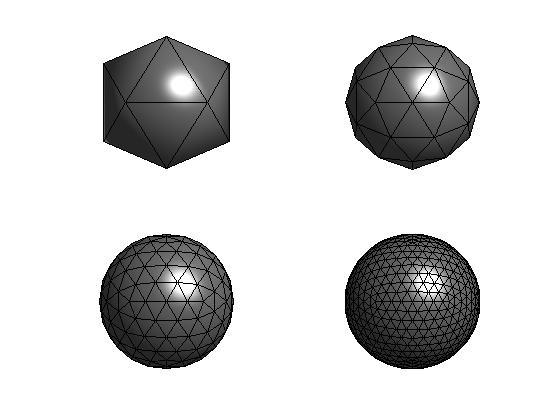Comput the center of each face.

x = [];
for i=1:3
v = vertex{end}(i,:);
x(i,:) = mean(v(face{end}));
end


name = 'lena';
M = rescale( load_image(name, 512) );


Display it.

clf;
imageplot(crop(M));Load a function on the sphere. Use the center of each face to sample the function.

f = rescale( load_spherical_function(M, x, options) );


Display the function on the sphere.

vv = [125,-15];
options.face_vertex_color = f;
clf;
plot_mesh(vertex{end}, face{end}, options);
view(vv);
colormap gray(256);
lighting none;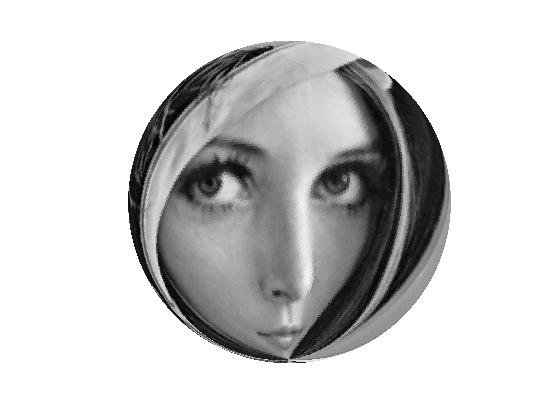## Multiscale Low Pass

One can compute low pass approximation by iteratively averaging over 4 neighboring triangles.

Perform one low pass filtering.

f1 = mean( reshape(f, [length(f)/4 4]), 2);


Display.

clf;
options.face_vertex_color = f1;
plot_mesh(vertex{end-1}, face{end-1}, options);
view(vv);
lighting none;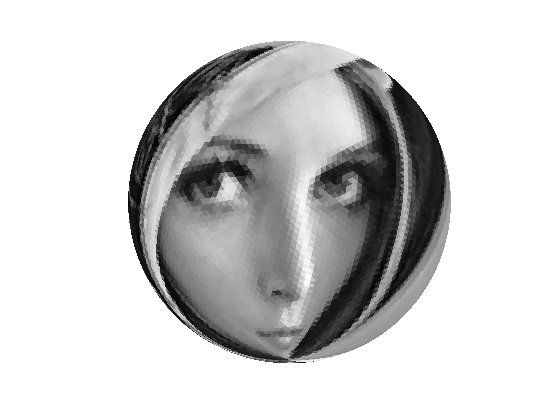Exercice 1: (check the solution) Compute the successive low pass approximations.

exo1;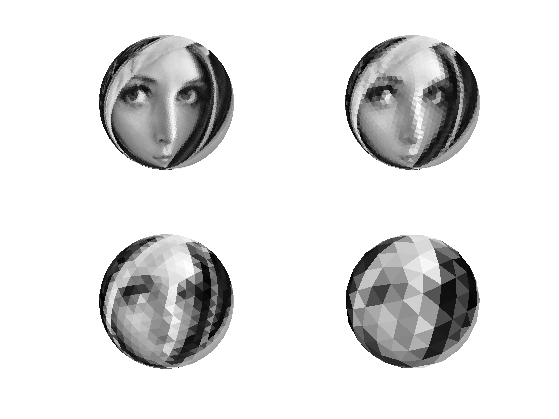## Spherical Haar Transform

One can compute a wavelet transform by extracting, at each scale, 3 orthogonal wavelet coefficient to represent the orthogonal complement between the successive resolutions.

Precompute the local wavelet matrix, which contains the local vector and three orthognal detail directions.

U = randn(4);
U(:,1) = 1;
[U,R] = qr(U);


Initialize the forward transform.

fw = f;
nj = length(f);


Extract the low pass component and apply the matrix U

fj = fw(1:nj);
fj = reshape(fj, [nj/4 4]);
fj = fj*U;


Store back the coefficients.

fw(1:nj) = fj(:);
nj = nj/4;


Exercice 2: (check the solution) Compute the full wavelet transform, and check for orthogonality (conservation of energy).

exo2;

Orthogonality deviation (should be 0): 5.5081e-15


Display the coefficients "in place".

clf;
options.face_vertex_color = clamp(fw,-2,2);
plot_mesh(vertex{end}, face{end}, options);
view(vv);
colormap gray(256);
lighting none;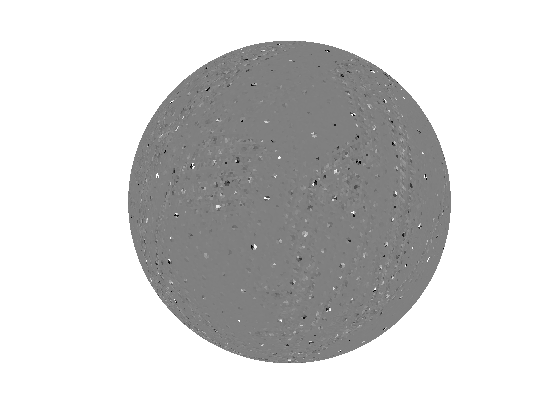Display the decay of the coefficients.

clf;
plot(fw);
axis([1 n -5 5]);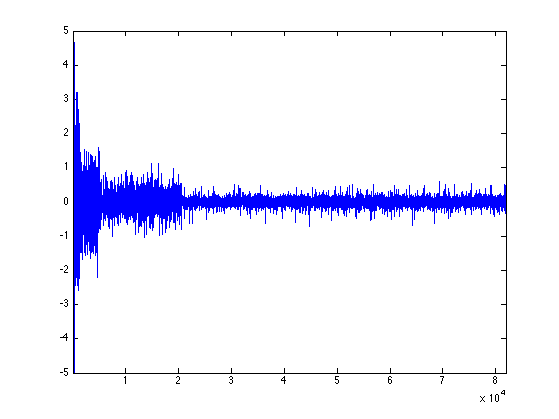Exercice 3: (check the solution) Implement the backward spherical Haar transform (replace U by U' to perform the reconstruction), and check for perfect reconstruction.

exo3;

clf;
options.face_vertex_color = clamp(f1);
plot_mesh(vertex{end}, face{end}, options);
view(vv);
colormap gray(256);
lighting none;

Bijectivity deviation (should be 0): 4.0915e-16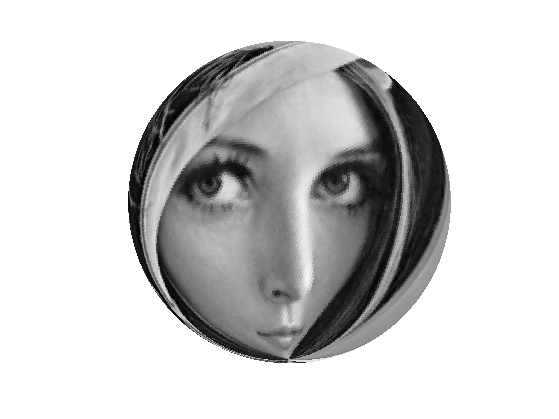Exercice 4: (check the solution) Perform Haar wavelet approximation with only 10% of the coefficients.

exo4;Exercice 5: (check the solution) Compare with the traditional 2D Haar approximation of M.

exo5;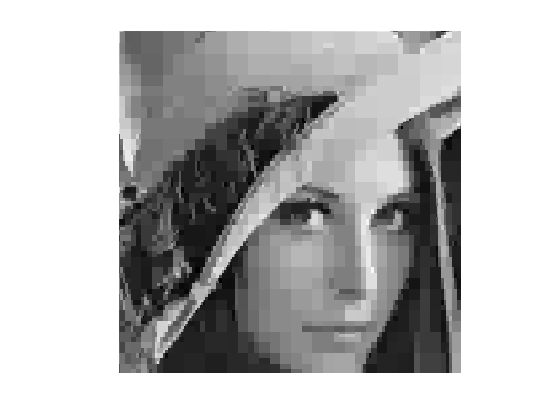Exercice 6: (check the solution) Implement Spherical denoising using the Haar transform. Compare it with vertex-based lifting scheme denoising.

exo6;